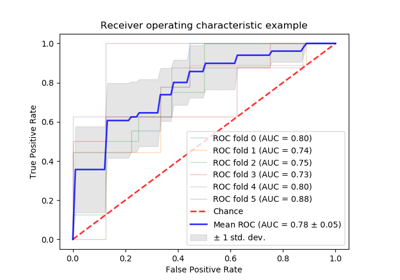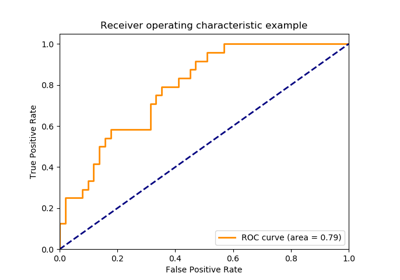# sklearn.metrics.roc_auc_score¶

sklearn.metrics.roc_auc_score(y_true, y_score, average='macro', sample_weight=None, max_fpr=None)[source]

Compute Area Under the Receiver Operating Characteristic Curve (ROC AUC) from prediction scores.

Note: this implementation is restricted to the binary classification task or multilabel classification task in label indicator format.

Read more in the User Guide.

Parameters: y_true : array, shape = [n_samples] or [n_samples, n_classes] True binary labels or binary label indicators. y_score : array, shape = [n_samples] or [n_samples, n_classes] Target scores, can either be probability estimates of the positive class, confidence values, or non-thresholded measure of decisions (as returned by “decision_function” on some classifiers). For binary y_true, y_score is supposed to be the score of the class with greater label. average : string, [None, ‘micro’, ‘macro’ (default), ‘samples’, ‘weighted’] If None, the scores for each class are returned. Otherwise, this determines the type of averaging performed on the data: 'micro': Calculate metrics globally by considering each element of the label indicator matrix as a label. 'macro': Calculate metrics for each label, and find their unweighted mean. This does not take label imbalance into account. 'weighted': Calculate metrics for each label, and find their average, weighted by support (the number of true instances for each label). 'samples': Calculate metrics for each instance, and find their average. Will be ignored when y_true is binary. sample_weight : array-like of shape = [n_samples], optional Sample weights. max_fpr : float > 0 and <= 1, optional If not None, the standardized partial AUC  over the range [0, max_fpr] is returned. auc : float

See also

average_precision_score
Area under the precision-recall curve
roc_curve
Compute Receiver operating characteristic (ROC) curve

References

  Fawcett T. An introduction to ROC analysis[J]. Pattern Recognition Letters, 2006, 27(8):861-874.

Examples

>>> import numpy as np
>>> from sklearn.metrics import roc_auc_score
>>> y_true = np.array([0, 0, 1, 1])
>>> y_scores = np.array([0.1, 0.4, 0.35, 0.8])
>>> roc_auc_score(y_true, y_scores)
0.75


## Examples using sklearn.metrics.roc_auc_score¶Receiver Operating Characteristic (ROC) with cross validationReceiver Operating Characteristic (ROC)